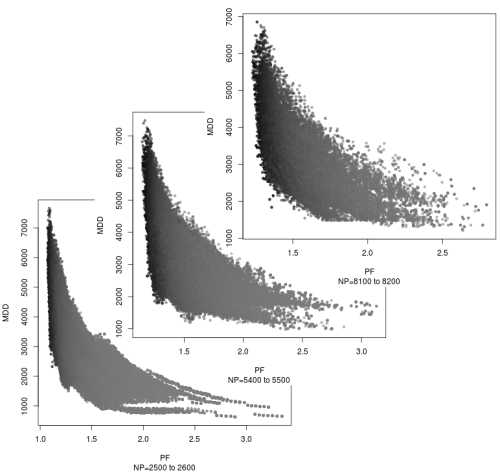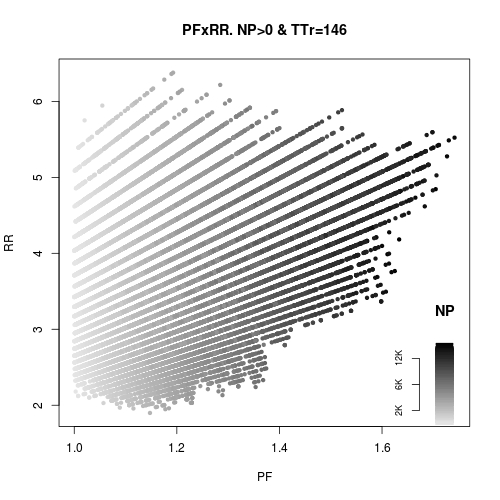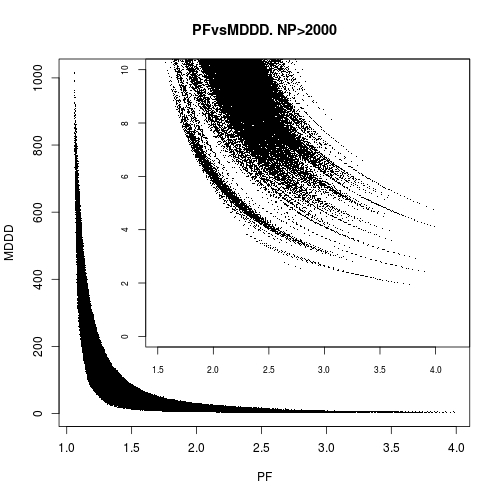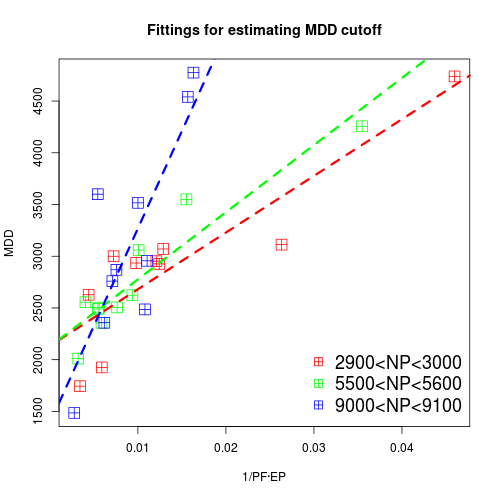Introduction

In the first part of this series we have seen the starting point of the development of an strategy and some of the performance statistics that can be useful in this early state of work. Some criteria for 'interesting' results have been discussed as stone marks for deciding if it is worth continuing the work or not.

In this part we discuss how this performance data with some other statistics can guide us in the next step, trying to understand the (possible) behavior of the system in the market.

First performance data analysis

I think that the most important information that we need at this stage of work is to understand the risks of our strategy. The reason is that I only find acceptable systems with contained and well understood loses, which will allow us to trade with confidence. This is dependent, of course, on the money management policy employed, but aiming first at the simple method of scaling leverage to our risk tolerance, we can think that the rough performance estimates obtained at this moment are appropriate proxies for real trade results.

So with this framework in mind, we start looking at some statistics from our tests:

Profit factor(PF): This statistic can be very informative. It signals how we are exploiting the (supposedly) edge we have over the market; to show how I present this plotting (obtained, as the rest in this article, from an exhaustive run of simulations in parametric space -more on this later- of Reverse Cyclop for EURUSD in the period from December 2011 to July 2012.):Plots of PF×MDD for very tight NP bands.

Here we can see a plot of PF×MDD for three very tight bands of NP performance (100&euro; gray intensity of the dots signals Total Trades (TTr), the darker the point the greater the number. We can see that PF correlates inversely to MDD and TTr; also that MDD has a clear lower cutoff, so there is a point where greater PFs do not improve MDD; we can see this graphics as a dynamic response of the system; given a NP performance with a 'bad' PF, we can think that if we can improve the PF, maintaining the NP we will improve, sometimes greatly, the MDD.

There is an optimal PF? Indeed:Plot of PF×NP for a very tight band at MDD cutoff, NP/MDD>1.

In this plot of PF×NP in a tight band at the MDD cutoff (200&euro, with points coding TTr in gray intensity as in the above graphs, we can see that maximum NP is in an small interval around PF=1.75; it is no coincidence (more on this later) that at roughly that number starts the MDD cutoff in the PF×MDD graphs. So we can infer that there is a maximum PF, not too high, that maximizes NP with the best NP/MDD ratios. Moreover, appears that there is also an optimal TTr, looking at the gray intensities in both graphs. To explore this idea we can look at the following plot:Plot of TTr×NP for three PF bands, MDD coded in color brightness.

Here we see TTr×NP for three PF bands represented by mark color, its brightness encoding MDD following the scale plotted in the lower right corner. The gaps introduced in PF between bands are on purpose, to avoid a color clutter in the picture. We see clearly an approximately circular region, centered on TTR=150,NP=12000 that maximizes NP with fairly good MDDs, with a radius of about 25TTr. This circle is the performance zone where we want to arrive, and achieving it is our ultimate milestone. With that idea we can trace the following method to perform tests that will allow us to extract a good first impression of performance versus risk:
• Do various simulations with parametric sets situated very sparsely in the parametric phase space, trying to pick a good fraction with NP>0, and add some of your best simulations obtained before; the idea is to find the TTr range and to have some starting good performing simulations for the following steps.
• After a convincing estimation of the TTr range, select a run with NP>0 and the best NP/MDD ratio. Looking at its PF and TTr we can think that: if PF is too low and TTr is in the upper part of its range, probably we can enhance the results decreasing TTr. So the idea is to modify the suitable parameters (the fewer the better) that tighten the trading rate and to look what happens. Inversely, if PF is too high and TTr goes in the lower part of its range, loosening the trading rate and picking more signals probably will enhance the result also. If we get a case with bad PF and central TTr, we can think that we are exiting the market too early and/or too lately; PF is correlated to Reward-to-Risk(RR) ratio as can be seen in the plot below, so increasing the RR will possibly enhance the results; modify the SL and TP computation, nearing the former and moving further away the latter and look what happens. Iterate this process more times with other starting runs to cover as exhaustively as possible the pertinent results subspace spanned by PF×TTr.Plot of PF×RR for TTr=146, NP coded in gray brightness.

• After we have a good idea of where is situated (at least roughly) the optimal PF and TTr, is time to estimate the MDD cutoff. This way we can limit the possible NP/MDD ratios and acquire a good lower limit on risk performance, which will allow us later to adjust the money management policy. To do it we can use MDDD, as can be seen in the plot of PF×MDDD below; there we can see that MDDD is related to PF roughly by an x-1 law, so making use of it we have that MDDD∝(PF×EP)-1. With the data that we have at this stage, the best we can do is to make linear fittings for this proportionality relation in tight NP bands, as can be seen in the graph below. The point of cross at 0 of each fitting is our MDD cutoff estimation, which in general we can take as an high estimate.Plot of PF×MDDD for TTr=146, with a zoomed inset at the most interesting region.Plot of linear fittings for estimating MDD cutoff in three random samples of 10 simulations within three different tight NP bands.

• Now we can take the best NP in the optimal PF area and the best MDD cutoff obtained in the linear fittings and take its NP/MDD ratio as an high estimate of the best NP/MDD ratio that we can obtain in the MDD cutoff line at the optimal PF. Calculate a WSR at the MDD cutoff computing an Average Win with the mean of the win percents of the best simulations at the optimal PF area and the best NP taken above. Finally a MDDD at the MDD cutoff using as EP the one obtained from the best NP we are using divided by the optimal TTr estimated. Then we have now high estimators of the best MDD, MDDD, WSR and NP/MDD that we can expect to have at this stage of development.

Deciding to continue the work

With this risk estimates we can see clearly if the system has a possible broad edge over the market or not, and if the profit is worth the risk. All the above computations can be done in little time, either from a simulator as my C++ Simulator, or a first working code in JForex, and can avoid lengthly periods of testing using more traditional optimization techniques.

Recomendation: At this stage is a must to have fairly good numbers both in performance and risk metrics. TTr must be in a band between about 2% and 8% of the total number of candles of reference (for example, the candle of reference that I am using in Reverse Cyclop with EURUSD is 1H) in the period tested. Above that band we are almost surely overtrading. A clear traced line require an NP/MDD>2, WSR<10, MDDD less than half the TTr, and an EP clearly capable of absorbing trading commissions, price slippage and spread, leaving additionally some profit. Anything nonperforming like these most probably will not produce a solid system, and ultimately will not pass the test of the market, so the (small) possibilities to success are not worth the time and money that we will spend if we continue at this point.

Now, if we decide to continue the development of the strategy, is time to gauge its behavior in a more real trading environment, which is where we will continue in the next part of this series. We will see how to decide a good money management policy to perform market tests and to which statistics we have to take attention to guide our work.

Translate to English Show original